@Team 2018-12-08T04:06:06.000000Z 字数 4232 阅读 1668

# 在pytorch、keras中自由使用tensorboard的简单方案

刘威威

## tensorboard类的实现

Tensorboard提供以下基本功能：

• 可视化Tensorflow图
• 绘制一个简单的值（如学习率）
• 绘制图像（例如激活图）
• 绘制直方图。

import ioimport numpy as npfrom PIL import Imageimport tensorflow as tfimport skimageimport matplotlibmatplotlib.use("TkAgg")import matplotlib.pyplot as pltclass Tensorboard:    def __init__(self, logdir):        self.writer = tf.summary.FileWriter(logdir)    def close(self):        self.writer.close()    def log_scalar(self, tag, value, global_step):        summary = tf.Summary()        summary.value.add(tag=tag, simple_value=value)        self.writer.add_summary(summary, global_step=global_step)        self.writer.flush()    def log_histogram(self, tag, values, global_step, bins):        counts, bin_edges = np.histogram(values, bins=bins)        hist = tf.HistogramProto()        hist.min = float(np.min(values))        hist.max = float(np.max(values))        hist.num = int(np.prod(values.shape))        hist.sum = float(np.sum(values))        hist.sum_squares = float(np.sum(values ** 2))        bin_edges = bin_edges[1:]        for edge in bin_edges:            hist.bucket_limit.append(edge)        for c in counts:            hist.bucket.append(c)        summary = tf.Summary()        summary.value.add(tag=tag, histo=hist)        self.writer.add_summary(summary, global_step=global_step)        self.writer.flush()    def log_image(self, tag, img, global_step):        s = io.BytesIO()        Image.fromarray(img).save(s, format='png')        img_summary = tf.Summary.Image(encoded_image_string=s.getvalue(),                                       height=img.shape,                                       width=img.shape)        summary = tf.Summary()        summary.value.add(tag=tag, image=img_summary)        self.writer.add_summary(summary, global_step=global_step)        self.writer.flush()    def log_plot(self, tag, figure, global_step):        plot_buf = io.BytesIO()        figure.savefig(plot_buf, format='png')        plot_buf.seek(0)        img = Image.open(plot_buf)        img_ar = np.array(img)        img_summary = tf.Summary.Image(encoded_image_string=plot_buf.getvalue(),                                       height=img_ar.shape,                                       width=img_ar.shape)        summary = tf.Summary()        summary.value.add(tag=tag, image=img_summary)        self.writer.add_summary(summary, global_step=global_step)        self.writer.flush()if __name__ == '__main__':    tensorboard = Tensorboard('logs')    x = np.arange(1, 101)    y = 20 + 3 * x + np.random.random(100) * 100    # Log simple values    for i in range(0, 100):        tensorboard.log_scalar('value', y[i], i)    # Log images    img = Image.open('/mnt/sda1/lvv/84504.jpg')    img = np.asarray(img, dtype=np.uint8)    # img = skimage.io.imread('/mnt/sda1/lvv/84504.jpg')    tensorboard.log_image('example_image', img, 0)    # Log plots    fig = plt.figure()    plt.plot(x, y, 'o')    tensorboard.log_plot('example_plot', fig, 0)    plt.close()    # Log histograms    rng = np.random.RandomState(10)    a = np.hstack((rng.normal(size=1000), rng.normal(loc=5, scale=2, size=1000)))    tensorboard.log_histogram('example_hist', a, 0, 'auto')    tensorboard.close()

log_scalar，log_image，log_plot和log_histogram函数都将tag和global_step作为参数。 tag是要绘制的值的任意名称。 例如，你可以为loss函数设置tag ='loss'。 global_step指的是测量特定值的时间，例如时期计数或类似值。 此外，log_scalar接受数值作为其第二个参数。 这是log_scalar生成的示例标量图：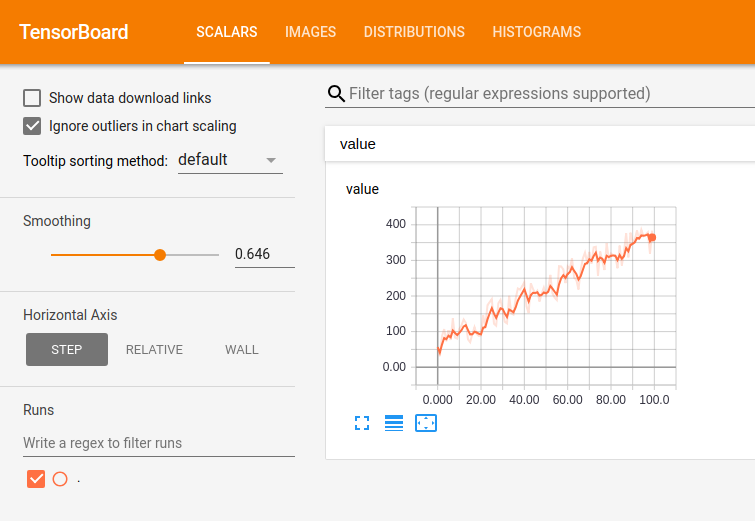log_image是一个类似的简单函数，它将一个numpy数组作为img参数来绘制任意图像（例如下面的例子）：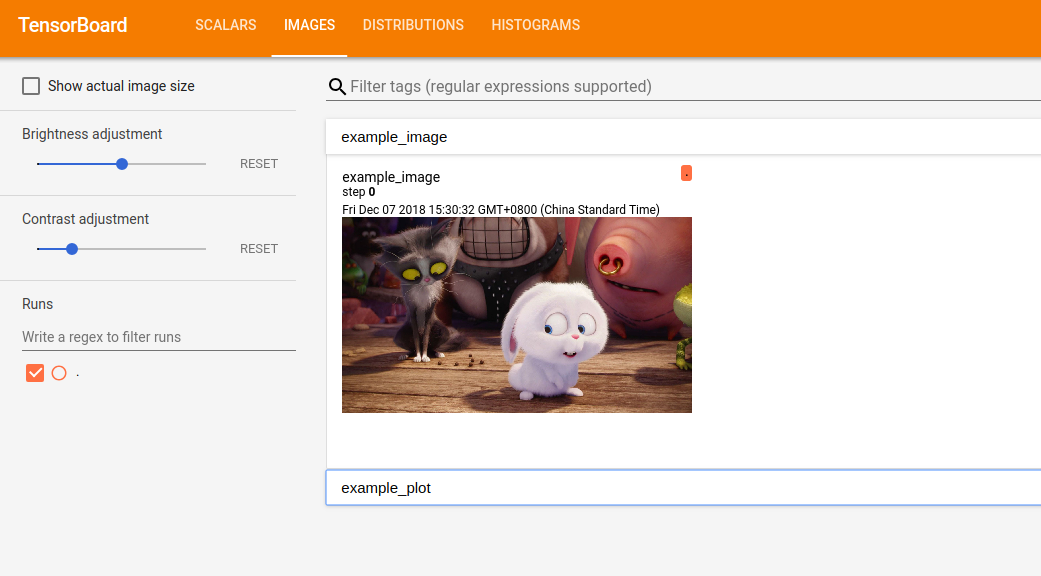log_histogram稍微复杂一些：它使用bin的bin数来计算values参数中给出的值的直方图。 计算本身就是numpy。 然后，它被送到Tensorboard：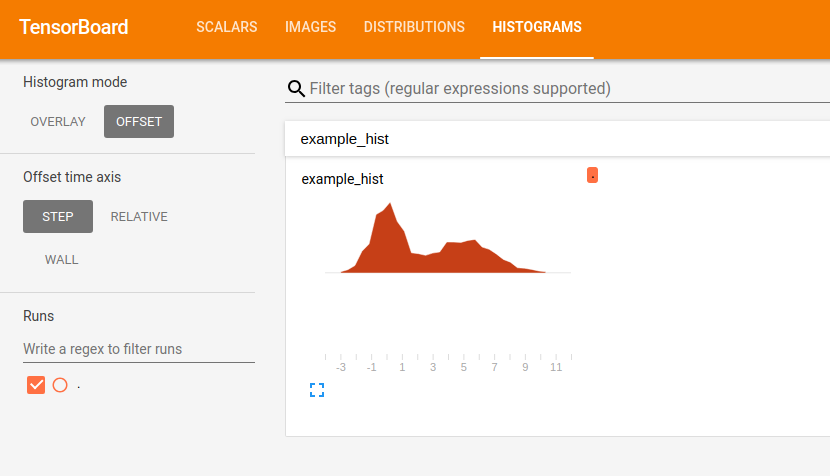log_plot与log_image非常相似，只是提供的输入不是image，它的figure参数需要是一个matplotlib图。 使用此函数，你可以直接在Tensorboard中显示任意matplotlib figures ：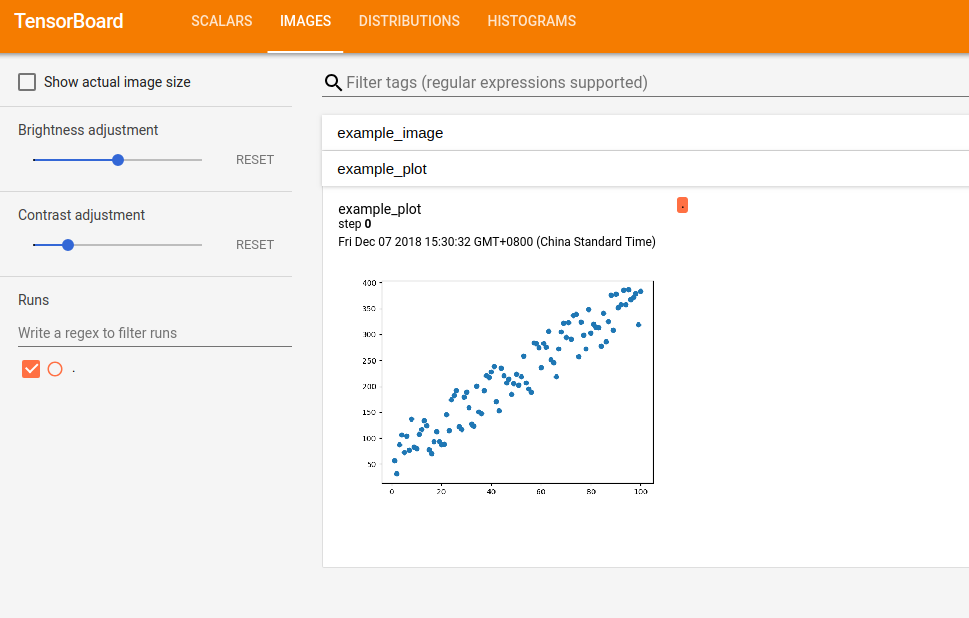## 使用tensorboard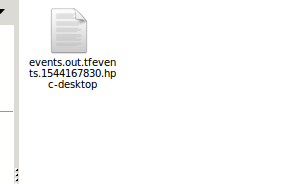$tensorboard --logdir=logs  如果出现错误，端口不可用等情况，可以指定port参数或者host参数等，参考示例： $tensorboard --logdir=logs  --host=127.0.01  --port=8008

\$tensorboard -h• 私有
• 公开
• 删除